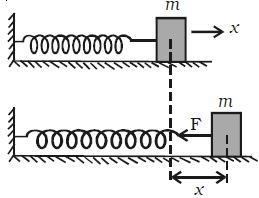# Horizontal Oscillations of Spring

Consider a mass (m) attached to an end of a spiral spring (which obeys Hooke’s law) whose other end is fixed to a support as shown in Figure. The body is placed on a smooth horizontal surface. Let the body be displaced through a distance x towards right and released. It will oscillate about its mean position. The restoring force acts in the opposite direction and is proportional to the displacement.Restoring force F = – kx.

From Newton’s second law, we know that F = ma

So, ma = – kx

So, a = (-k/m)*x

Comparing with the equation of simple harmonic motion, a = – ω2x

We get, ω2 = (k/m)

Or, ω = √( k/m)

But, T = 2π/ω

Time period = T = 2π √( m/k)

And frequency, n = 1/T = 1/2π [√( k/m)]